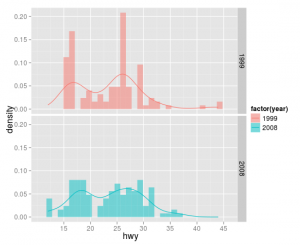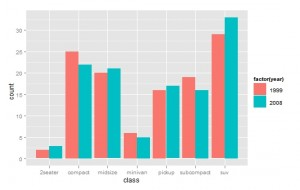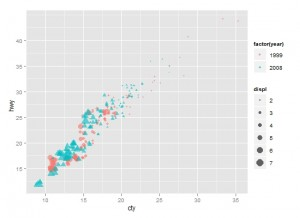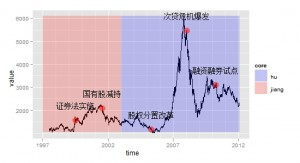420,748

# I. 导论

1 什么是ggplot2

ggplot2是用于绘图的R语言扩展包，其理念根植于《Grammar of Graphics》一书。它将绘图视为一种映射，即从数学空间映射到图形元素空间。例如将不同的数值映射到不同的色彩或透明度。该绘图包的特点在于并不去定义具体的图形（如直方图，散点图），而是定义各种底层组件（如线条、方块）来合成复杂的图形，这使它能以非常简洁的函数构建各类图形，而且默认条件下的绘图品质就能达到出版要求。

2 与lattice包的比较

ggplot2和lattice都属于高级的格点绘图包，初学R语言的朋友可能会在二者选择上有所疑惑。从各自特点上来看，lattice入门较容易，作图速度较快，图形函数种类较多，比如它可以进行三维绘图，而ggplot2就不能。ggplot2需要一段时间的学习，但当你跨过这个门槛之后，就能体会到它的简洁和优雅，而且ggplot2可以通过底层组件构造前所未有的图形，你所受到的限制只是你的想象力。

3 基本概念

• 图层（Layer）：如果你用过photoshop，那么对于图层一定不会陌生。一个图层好比是一张玻璃纸，包含有各种图形元素，你可以分别建立图层然后叠放在一起，组合成图形的最终效果。图层可以允许用户一步步的构建图形，方便单独对图层进行修改、增加统计量、甚至改动数据。
• 标度（Scale）：标度是一种函数，它控制了数学空间到图形元素空间的映射。一组连续数据可以映射到X轴坐标，也可以映射到一组连续的渐变色彩。一组分类数据可以映射成为不同的形状，也可以映射成为不同的大小。
• 坐标系统（Coordinate）：坐标系统控制了图形的坐标轴并影响所有图形元素，最常用的是直角坐标轴，坐标轴可以进行变换以满足不同的需要，如对数坐标。其它可选的还有极坐标轴。
• 位面（Facet）：很多时候需要将数据按某种方法分组，分别进行绘图。位面就是控制分组绘图的方法和排列形式。

4 一个例子

[code lang="R"]
library(ggplot2)
p <- ggplot(data=mpg,aes(x=displ,y=hwy,colour=factor(cyl)))
p + geom_point() + geom_smooth()
[/code]

`上图是对几种不同汽缸的数据分别平滑，如果需要对整体数据进行平滑，可将colour参数设置在散点图层内而非第一层，这样第三层的平滑图形就不会受到colour参数的影响。`

[code lang="R"]
p <- ggplot(mpg,aes(x=displ,y=hwy))
p + geom_point(aes(colour=factor(cyl))) + geom_smooth()
[/code]

# II. 图层控制与直方图

ggplot2使用图层将各种图形元素逐步添加组合，从而形成最终结果。第一层必须是原始数据层，其中data参数控制数据来源，注意数据形式只能是数据框格式。aes参数控制了对哪些变量进行图形映射，以及映射方式，aes是Aesthetic的缩写。

library(ggplot2)
[code lang="R"]
p <- ggplot(data = mpg,aes(x = hwy))
p <- p + geom_histogram()
summary(p)
[/code]

```
data: manufacturer, model, displ, year, cyl, trans,
drv, cty, hwy, fl, class [234x11]
mapping:  x = hwy
faceting: facet_grid(. ~ ., FALSE)
-----------------------------------
geom_histogram:
stat_bin:
position_stack: (width = NULL, height = NULL)```

[code lang="R"]
p <- ggplot(mpg,aes(hwy))
p + geom_histogram(position = 'identity',
alpha=0.5,
aes(y = ..density..,
fill = factor(year))) +
stat_density(geom = 'line',
position = 'identity',
aes(colour = factor(year)))
[/code]

`如果想将两个直方图分开绘制，也可以使用facet_grid参数，结果如下图所示。`

# III. 位置调整与条形图

[code lang="R"]
library(ggplot2)
with(mpg,table(class,year))
p <- ggplot(data=mpg,aes(x=class,fill=factor(year)))
p + geom_bar(position='dodge')
p + geom_bar(position='stack')
p + geom_bar(position='fill')
p + geom_bar(position='identity',alpha=0.3)
[/code]geom_bar是绘制条状几何对象，所以也可以用不经汇集的原始数据进行绘图。下面我们用2001到2010年间的美国GDP增长率举个例子。
[code lang="R"]
y=c(1.1,1.8,2.5,3.6,3.1,2.7,1.9,-0.1,-3.5,3.0)
x=2001:2010
data=data.frame(x,y)
p=ggplot(data,aes(x,y,fill=y))
p+geom_bar(stat="identity")+
geom_abline(intercept = 0, slope = 0,size=1,colour='gray')+
geom_text(aes(label=y),hjust=0.5, vjust=-0.5 )+
scale_y_continuous(limits=c(-3.8,4.2))+
labs(x='年份', y='GDP增长率%')+
opts(title = "美国GDP增长率")
[/code]

# IV. 散点图

1 色彩和形状的控制

[code lang="R"]
library(ggplot2)
p <- ggplot(mpg, aes(cty, hwy))
p1 <- p + geom_point(aes(colour = factor(year),shape = factor(year), size = displ), alpha = 0.6, position = 'jitter')
print(p1)
[/code]2 坐标的控制

[code lang="R"]
cty.mean=with(mpg,mean(cty))
cty.sd=with(mpg,sd(cty))
p1 + scale_x_continuous(trans='log',breaks=c(cty.mean-cty.sd,cty.mean,cty.mean+cty.sd), labels=c("high", "mean", "low")) + scale_y_continuous(trans='log')
[/code]
3 文字说明

[code lang="R"]
p <- ggplot(mtcars, aes(x=wt, y=mpg,colour=factor(cyl),label=rownames(mtcars)))
p + geom_text(hjust=0,vjust=-1,alpha=0.8)+ geom_point(size=3,aes(shape=factor(cyl)))
[/code]

4 矩阵散点图

ggplot2包中也提供了矩阵散点图函数
[code lang="R"]
plotmatrix(USArrests)+geom_smooth()
[/code]

# V. 时间序列

ggplot2包也能对时间序列数据绘图，但在处理上需要有些注意的地方。下面我们以上证指数为例进行作图，首先利用quantmod包从yahoo数据源获取从1997年以来的数据，存于变量SSEC中，抽取收盘数字，然后分别提取时间数据和指数数值，绘图结果如下图。

[code lang="R"]
library(quantmod)
library(ggplot2)
getSymbols('^SSEC',src='yahoo',from = '1997-01-01')
close <- (Cl(SSEC))
time <- index(close)
value <- as.vector(close)
p <- ggplot(data.frame(time,value),aes(time,value))
p + geom_line()
[/code]

[code lang="R"]
yrng <- range(value)
xrng <- range(time)
data <- data.frame(start=as.Date(c('1997-01-01','2003-01-01')),end=as.Date(c('2002-12-30','2012-01-20')),core=c('jiang','hu'))
timepoint <- as.Date(c('1999-07-02','2001-07-26','2005-04-29','2008-01-10','2010-03-31'))
events <- c('证券法实施','国有股减持','股权分置改革','次贷危机爆发','融资融券试点')
data2 <- data.frame(timepoint,events,stock=value[time %in% timepoint])
[/code]

[code lang="R"]
p + geom_line()
+ geom_rect(aes(NULL,NULL,xmin = start, xmax = end, fill = core),ymin = yrng,ymax=yrng,data = data)
+ scale_fill_manual(values = alpha(c('blue','red'),0.2))
+ geom_text(aes(timepoint, stock, label = events),data = data2,vjust = -2,size = 5)
+ geom_point(aes(timepoint, stock),data = data2,size = 5,colour = alpha('red',0.5))
[/code]`参考资源：`

http://xccds1977.blogspot.com

ggplot2 Elegant Graphics for Data Analysis （下载

• 本文由 发表
• 转载请务必保留本文链接：https://www.plob.org/article/3553.html

• cionysus 0

感谢分享！

• zhuwen96485 1

感谢楼主分享